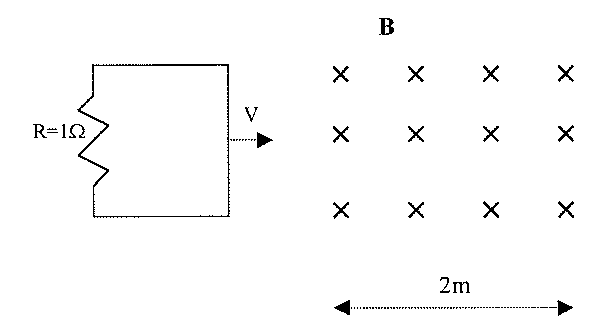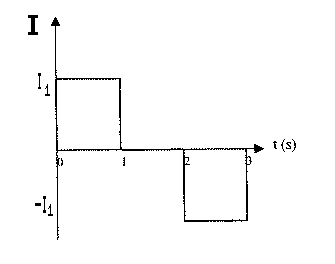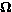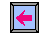## University of California, San Diego Physics 1b - Thermal Physics & Electromagnetism

 H. E. Smith Spring 2000Physics 1B - Tutorial #101. The figure above shows two coils, A & B. Use Lenz' Law to determine which direction current flows through the resistor when
• (a) the switch is closed.
• (b) the switch is opened after being closed.
• (c) the current in A decreases.
• (d) the current in A increases.
• (e) B is moved toward A.
In (a), (d), (e) the flux of magnetic field toward the right is increasing, by Lenz' Law B will act to oppose the increasing flux, by creating a flux/field in the opposite direction - current will be right to left.
In (b) & (c) current will be left to right.2. The conducting loop shown above has area A = 1m2 (1m on a side) and is moving with v=1m/s into a magnetic field B = 10-4T. Graph the current in the loop as a function of time.From Faraday's Law= -d/dt = B . dA/dt = Blv = 10-4T.1m.1m/s = 10-4V as the loop enters the field. If emf and current are positive counter-clockwise, the current will behave as shown on the left, with I1 = 10-4A.

3. An apartment complex is supplied by single-phase AC from a power plant 5-km away. The wire that runs from the power plant to the complex and back has a resistance per unit length of 1 x 10-5/m, supplying 100,000W.
• Calculate the resistive power losses in the wire if the AC is supplied at 110V.
The total wire resistance is 1 x 10-5 . (5000m + 5000m) = 0.1. The power supplied is P = VI so that I = 100,000W/110V = 910A. The power lost to heating the wire due to its resistance is P = I2R = (910A)2. 0.1= 83kW. In other words, the power plant has to produce 183kW in order to deliver 100kW, losing 45% to resistive heating.

• Calculate the resistive power losses in the wire if the AC is supplied at 500kV (typical of US electric distribution).
In this case the current is much lower, I = 100,000W/500,000V = 0.2A and the resistive losses, P = (0.2A)2. 0.1= 0.004W, are negligible.

• Design a way to transform AC provided at 500kV to 110V.
You need a transformer, one of those big cylinders that you see mounted on power poles which uses magnetic induction such that Vsource/Vhousehold = Ns/Nh, where N is the number of turns of wire on the appropriate side of the transformer. In this case, Vsource/Vhousehold = 500,000V/110V = 4545, so the turn ratio of this step-down transformer is Ns/Nh = 4545 (e.g. if the output - 110V - side of the transformer has 100 turns, the input side would need 454,545 turns).Physics 1B HomeTutorials

Gene SmithPhysics 1B HomeTutorialsTutorial #9